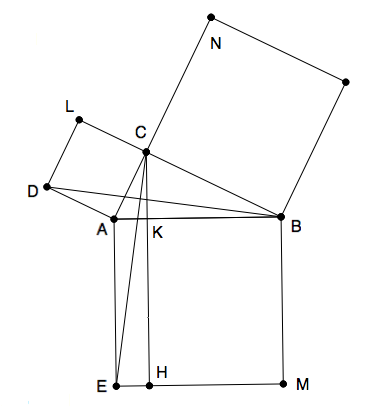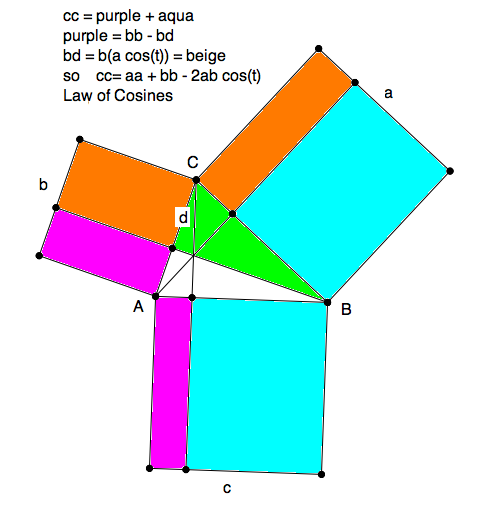newcommand("\pll","\u2227")
newcommand("\and","\u002e\u2227\u002e")
newcommand("\or","\u2228")

Lesson E4
Last edited 8feb15
Euclid's Elements end with Pythagoras

\textit{$\C$  2010, Prof. George K. Francis, Mathematics Department,
University of Illinois}

\begin{document}
\maketitle\section{Introduction}
In this lesson we will conclude our study Euclid's Elements, which end
with Euclid's proof of the Pythagoraen Theorem.

\section{The Theorem}
The original statment of Pythagoras' theorem is phrased geometrically, not
numerically, as we do today. It says that the square erected on the
hypotenuse of a right triangle can be decomposed into the sum of the two
squares built on the two sides of the triangle. There is no hint of the
formula you learned in high school $a^2 + b^2 = c^2$, even if you identify
the variables properly as the lengths of the sides of a right triangle.
The theorem was known and used millenia before Euclid or even Pythagoras. The Greeks
are credited with developing an aximatic system that leads to a rigorous
proof of the theorem.

\section{Euclid's Proof}
There are many proofs of this theorem, and it makes good sense to build
an entire high school geometry course about all the various proofs. Here,
however, we will study just a few  of them, for their contrasting
geometrical import. First, Euclid's proof. We avoid using letters to
refer to points and lines on purpose. It helps you study the figure. In
your Journal you should draw a figure, label it, and then \textbf{use}
your lettering in the proof. This makes it easier to follow the proof.

Question 1.

Locate, on the WWW or a book,  a proof different from the one here.
Study it and put it into your Journal. Here, cite the reference where
you obtained the proof, and a (very) short description.

Given is a right triangle with  three squares on its sides. The largest
square is on the hypotenuse, and the smallest and middling squares are
on the legs, unless the triangle is isosceles. You should avoid
using isosceles right triangles. Although the theorem remains true
for these, the proof requires editing.

We shall prove that one of these rectangles, say the smaller, has the
same area as the smaller square on the sides of the right triangle.
Drop the altitude to the hypotenuse and continue it through the largest
square, dissecting it into two unequal rectangles.
The altitude also divided the given right triangle into two
smaller right triangles.  Adding the smaller right triangle to the smaller
of the rectangles yields a right angled trapezoid
with parallel sides equal to the the extended altitude and the extended
side of the largest square.

The longer diagonal of this trapezoid makes an obtuse triangle based
on the side of of the largest square.
We claim that this obtuse triangle has half the area of
the small rectangle.

Today we would just quote that the area of a triangle is half the base
times the height, which are also the base and height of the rectangle.
Euclid would divide the rectangle in half, using the diagonal of the
rectangle, and then claim the area of the two triangles to be equal.

Question 2.

Identify which Proposition (after the 29th) justifies the claim that
the obtuse $\triangle ABD$ has the same area as $\triangle ACD$.

The same reasoning applies to another obtuse triangle formed by the
diagonal of a trapezoid with base the side of the smallest square.
The two obtuse triangles have two sides pairwise equal to each other,
and the two (obtuse) angles between the corresponding sides are equal
as well. This claim can be verified by decomposing the obtuse angle
into the sum of a right angle, and an acute angle of the original
right triangle.  This acute angle is common to both obtuse angles.
Therefore we can apply SAS to show that the two obtuse triangles
are congruent, and in particular, have the same area.

We'll call these \textit{ Euclid's Obtuse Triangles}. Note that
in a geometric construction package, like GEX or GGB,
you can do this differently. Rotate one of Euclid's obtuse
triangles into the other by a 90 degree rotation.

Question 3.

Did you completer the construction rotating one of Euclid's obtuse
triangles into the other?

We have  shown that the square on the smaller side has the same area
as the smaller rectangle in the square on the hypotenuse. By
logical symmetry, the same argument applies to the other rectangle
in the square on the hypotenuse, and we are done proving the theorem.

\section{The Law of Cosines}Euclid's proof of Pythagoras' theorem uses a subtheorem, usually called
a \textit{lemma}, which generalizes to triangles which are \textbf{ not}
right triangles. We describe the acute case, and leave the obtuse case
as an exercise.

\subsection{The acute case}
\begin{itemize}
\item Relax the condition that the initial $\triangle ABC$
is a right triangle, and allow it to be an acute triangle.
\item We can still construct squares on each of the sides.
\item Drop and extend two altitudes, say from $C$ and $B$.
\item Apply Euclid's construction and argument (using SAS) to show that adjacent
rectangles have the same area.
\item This much shows that $cc = aa + bb - 2bd$.
\item It remains only to recognize the $d= a \cos( \angle ACB ))$.
\end{itemize}

Question 4.

Why is $d= a \cos( \angle ACB ))$ in the figure? You may use Euclid's
definition of the cosine as the ratio of the opposite leg to the hypotenuse
of a right triangle.

\subsection{The obtuse case}
In the case that $\triangle ABC$ has an obtuse angle at $C$, two of
the altitudes no longer cut the smaller squares into rectangles. In
fact, you need to extend the sides $b$ and $c$ for the altitudes to
reach them at all. Now, the squares $aa$ and $bb$ become part of larger
rectangles. This is to be expected, because for $\angle ACB$ obtuse,
its cosine is negative, so we must add, not subtract, rectangles to
the smaller squares in order to make up the area of the largest square.

Question 5.

Why can a triangle in Euclidean geometry have at most one obtuse angle?

\section{Problems}
The following problems will be assigned for solution elsewhere. You should
see how far you get the proofs and enter that into your Journal. Be sure you
leave opposite sides free for later ammendations and figures.

\textbf{Problem 1} Submit a proof, like the one above, but for obtuse
triangles. Include a screen shot of your figures.
Submit your .ggb or .gex file to permit the grader to check that

The following problems require working with the hyperbolic models of
non-Euclidean geometry  in GEX2.0 or GGB.
Answers should be based on experimentation, not on logical
deduction in Non-Euclidean geometry. You should work with one .gex
file, but edit screenshots of various cases to justify your assertions.
The .gex file and the the .pdf with the figures constitute a complete
solution. They are progressivley more difficult and you should consider
them a capstone, lab  exercise, worth extra credit.

\textbf{Problem 2} In a hyperbolic model of non-Euclidean geometry,
construct a counter example to Pythagoras' theorem. Recall that there
are no rectangles in Non Euclidean geometry.
You should use the Saccheri rectangles
as surrogate "squares" on the sides of a right triangle.
Then ask GEX or GGB to measure the areas of these surrogate "squares"
and thus show that Pythagoras' theorem is generally false. Is it ever true?

\textbf{Problem 3}
Drop the altitude to the hypotenuse and extend it. This cuts the